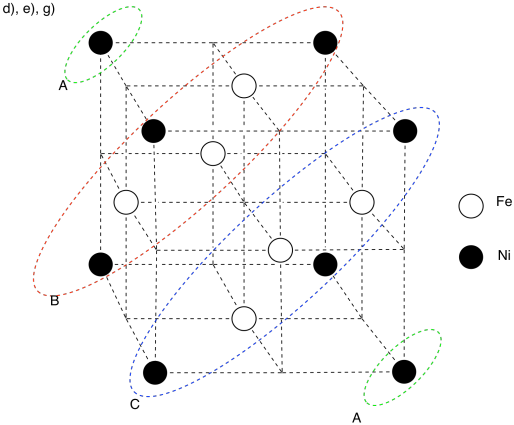# 2.4: Application Problems with Metals

Exercise $$\PageIndex{1}$$

Maraging steel is an alloy, Fe3Ni, that forms a face-centered cubic unit cell. It is used for high-strength applications such as in the forging of fencing swords (epées).

a) Draw a cube.

b) Add circles to denote atoms in a face-centered cubic array.
c) Calculate the number of atoms in the unit cell (always show work).

d) Shade in selected atoms in the unit cell to give a 3:1 ratio of iron:nickel in the unit cell.
e) Add a legend with shaded and non-shaded circles so that it is clear which circle represents which atom.

f) Face centered cubic can also be thought of as forming hexagonal layers. The layers alternate to give which pattern?

ABABAB or ABCABCABC

g) In the cubic diagram you made, put a letter beside each atom to indicate which of those layers (A or B or C) it is found in.

h) Why does the addition of nickel to the steel make the material more difficult to bend or break?There are eight atoms in the corner; each is shared by eight neighbouring cubes. There are six atoms on the faces; each is shared by two neighbouring cubes.

# atoms = $$8 (\frac{1}{8}) + 6 (\frac{1}{2}) = 1 + 3 = 4$$ atoms